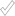CodingBat code practice

## Introduction to Mod Code

Video

This page introduces how the "mod" `%` operator works in Java and other languages along with a few live practice code problems.

## Mod % Operator

• Modulus operator % -- "mod" for short
• e.g. 23 % 10 → 3
• Repeatedly subtract 10 from 23 .. what's left?
• Like the "remainder" after dividing by 10
• Basically all languages use the % symbol for mod

You're familiar with the 4 arithmetic operations + - * /. The `%` modulus operator is an additional arithmetic operation: basically the remainder left over after division. For example, what is `73 % 10`? The simplest way to think about it is, keep subtracting 10's from 73 until there's less than 10 left (3 in this case).

• Mod yields 0 means divides evenly
•   e.g. 30 % 10 → 0
•   "N multiple of 5?" → (N % 5) == 0?

## Key Features of Mod

• When mod by N yields 0, N divides in evenly
• Mod by N yields a number in the range 0..N-1 (inclusive)
•   e.g. mod by 10 yields 0..9
•   e.g. mod by 100 yields 0..99
• Don't mod by 0, it's an error (like divide by 0 is an error)
• Don't use negative numbers ( * -1 as needed)

## Practice Mod Problemsor35 HspecialElevenold35more20less20nearTen

## MakeBricks Problem

More difficult problems that also use mod:makeBricksmakeChocolate

(in python:make_bricksmake_chocolate )